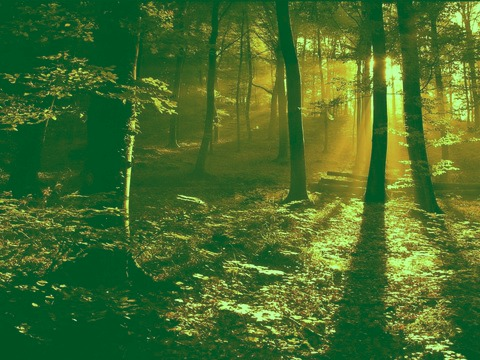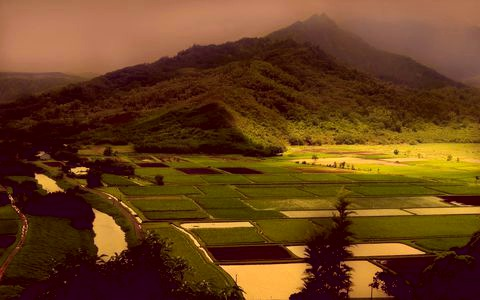## TRANSFERCOLOR

 Transfers the coloring from one image to another image.

 USAGE: transfercolor [-c colormode] [-p printcoefs] infile1 infile2 outfile USAGE: transfercolor [-h or -help] -c ... colormode ... colorspace mode of processing; options are: rgb, ..................... lab or ycbcr; default=lab -p ... printcoefs ... print the linear coefficients to the terminal; yes or no PURPOSE: To transfer the coloring of one image to another image. DESCRIPTION: TRANSFERCOLOR transfers the coloring from one image to another image. Specifically, the coloring of infile2 will be transfered to that of infile1. (infile1 will be colormatched to infile2). This is done via a linear transformation of each channel of the image after changing colorspace. The matching uses the mean and standard deviations from each channel according to the equation: (I2-Mean2)/Std2 = (I1-Mean1)/Std1. This equation represents an normalized intensity such that it has zero mean and approximately the same range of values due to the division by the standard deviations. We solve this equation to form a linear transformation between I1 and I2 according to I2=A*I1+B, where A=(Std2/Std1) is the slope or gain and B=(Mean2-A*Mean1) is the intercept of bias. ARGUMENTS: -c colormode ... COLORMODE is the colorspace mode of processing. The options are: rgb, lab or ycbcr. The default=lab. -p printcoefs ... PRINTCOEFS prints the linear coefficients to the terminal; options are yes or no. The default=no. CAUTION: This script may not be backward compatible prior to IM 6.8.6.0, when IM colorspace and grayscale changed. I have not tested it for earlier releases. Let me know if anyone tries and it fails. See http://www.imagemagick.org/discourse-server/viewtopic.php?f=4&t=21269 CAVEAT: No guarantee that this script will work on all platforms, nor that trapping of inconsistent parameters is complete and foolproof. Use At Your Own Risk.

### EXAMPLES

 Example 1 Image 1 (source)Image 2 (source)Arguments: -c labArguments: -c rgbArguments: -c ycbcrExample 2 Image 1 (source)Image 2 (source)Arguments: -c labWhat the script does is as follows: Converts both images to desired colorspace/channel Gets the mean and standard deviation from those images Computes the slope (gain) and intecept (bias) from the two sets Applies -function polynomial on each channel of the first image Recombines channels and convert back to RGB This is equivalent to the following IM commands convert -quiet "\$infile1" -colorspace \$colormode -separate +channel \$dir/tmpA.miff convert -quiet "\$infile2" -colorspace \$colormode -separate +channel \$dir/tmpB.miff (for ((i=0; i<3; i++)); do MA=`convert \$dir/tmpA.miff[\$i] -format "%[fx:mean]" info:` SA=`convert \$dir/tmpA.miff[\$i] -format "%[fx:standard_deviation]" info:` MB=`convert \$dir/tmpB.miff[\$i] -format "%[fx:mean]" info:` SB=`convert \$dir/tmpB.miff[\$i] -format "%[fx:standard_deviation]" info:` a=`convert xc: -format "%[fx:\$SB/\$SA]" info:` b=`convert xc: -format "%[fx:\$MB-\$a*\$MA]" info:` convert \$dir/tmpA.miff[\$i] -function polynomial "\$a \$b" miff:- done) | convert - -set colorspace \$colormode -combine -colorspace sRGB "\$outfile"